# Significance level

(diff) ← Older revision | Latest revision (diff) | Newer revision → (diff)
The probability of incorrectly rejecting the basic hypothesis being tested, when it is valid. In the theory of statistical hypotheses testing (cf. Statistical hypotheses, verification of), the significance level is also called the probability of an error of the first kind. The concept first arose in connection with the problem of testing for compatibility of a theory with experimental data. For example, suppose that observations are being conducted on the values ofrandom variablesand that, on the basis of these data, it is required to test a hypothesis, according to which the joint distribution ofhas some specific property. An appropriate statistical test is constructed with the aid of a suitably selected function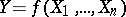; this function usually assumes small values whenis true, and large values whenis false. In particular, ifare the outcomes of independent measurements (with error) of some known constantand the hypothesisstates that no systematic errors are involved, then a reasonable choice ofis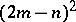, whereis the number of measured values of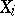that exceed the true value. A large observed value ofmay be considered a significant statistical refutation of the hypothetical agreement between the experimental outcome and the hypothesis. The corresponding significance test is a rule according to which values ofare considered significant if they exceed a prescribed critical value. In its turn, the choice ofis governed by the significance level, which equals the probability of the eventin the case that the hypothesisis true.
Selection of a significance level should also take into account the unavoidable errors incurred when any specific significance level is employed. For example, if the significance level is excessively high, the main error will stem from rejection of a true hypothesis; but if the significance level is low, the error will usually arise from accepting a false hypothesis. In practice, the most commonly adopted significance levels in statistical calculations range fromto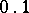. Significance levels lower than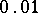are used, for example, in statistical detection of toxic medical preparates, and also in other special situations where the overriding purpose is to ensure against incorrect rejection of the hypothesis being tested. See also Confidence estimation.